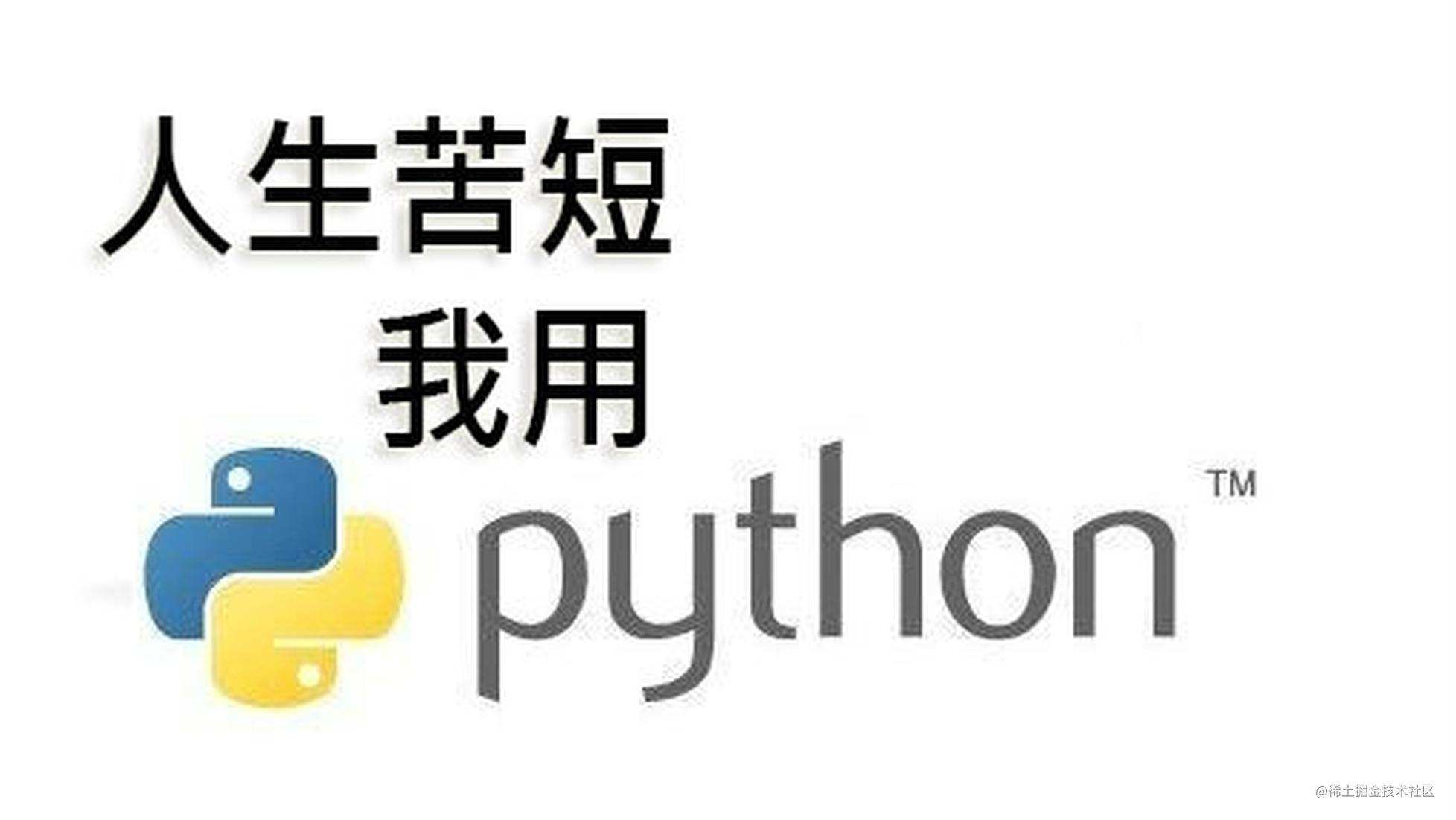# 详解 Python 模板引擎工作机制### 简介

``````from tornado import template
PAGE_HTML = """

Hello, { { username } }!

{ % for job in job_list % }
{ { job } }
{ % end % }

"""
t = template.Template(PAGE_HTML)

### 详解

``deftemplate_engine(template_string, **context):复制代码``

### 编译

``````def parse_template(template_string):
return python_source_code复制代码``````

``````
Hello, { { username } }!

{ % for job in jobs % }
{ { job.name } }
{ % end % }

复制代码``````

``````def _execute():
_buffer = []
_buffer.append('\n\n  Hello, ')
_buffer.append(str(_tmp))
_buffer.append('!\n  \n    ')
for job in jobs:
_buffer.append('\n      ')
_tmp = job.name
_buffer.append(str(_tmp))
_buffer.append('\n    ')
_buffer.append('\n  \n\n')
return''.join(_buffer)复制代码``````

``````_buffer.append('hello')
_append_buffer = _buffer.append
_append_buffer('hello')复制代码``````

`{ { ... } }` 中的表达式将会被提取出来，然后添加进 `string` 列表中。在 `tornado` 模板模块中，在 `{ { ... } }` 所编写的表达式没有任何的限制，iffor 代码块都可以准确地转换成为 Python 代码。

### 让我们来看看具体的代码实现吧

``````class Template(object):
def__init__(self, template_string):
self.code = parse_template(template_string)
self.compiled = compile(self.code, '', 'exec')复制代码``````

``````class _TemplateReader(object):
def __init__(self, text):
self.text = text
self.pos = 0
def find(self, needle, start=0, end=None):
pos = self.pos
start += pos
if end is None:
index = self.text.find(needle, start)
else:
end += pos
index = self.text.find(needle, start, end)
if index != -1:
index -= pos
return index
def consume(self, count=None):
if count is None:
count = len(self.text) - self.pos
newpos = self.pos + count
s = self.text[self.pos:newpos]
self.pos = newpos
return s
def remaining(self):
return len(self.text) - self.pos
def __len__(self):
return self.remaining()
def __getitem__(self, key):
if key < 0:
return self.text[key]
else:
return self.text[self.pos + key]
def __str__(self):
return self.text[self.pos:]复制代码``````

``````class _CodeWriter(object):
def __init__(self):
self.buffer = cStringIO.StringIO()
self._indent = 0
def indent(self):
return self
def indent_size(self):
return self._indent
def __enter__(self):
self._indent += 1
return self
def __exit__(self, *args):
self._indent -= 1
def write_line(self, line, indent=None):
if indent == None:
indent = self._indent
for i in xrange(indent):
self.buffer.write("    ")
print self.buffer, line
def __str__(self):
return self.buffer.getvalue()复制代码``````

`parse_template` 函数里，我们先要创建一个 `_TemplateReader` 对象：

``````def parse_template(template_string):
writer = _CodeWriter()
file_node.generate(writer)
return str(writer)复制代码``````

``````def _parse(reader, in_block=None):
body = _ChunkList([])
while True:
curly = 0
while True:
if curly == -1 or curly + 1 == reader.remaining():
if in_block:
raise ParseError("Missing { %% end %% } block for %s" %
in_block)
return body
if reader[curly + 1] not in ("{", "%"):
curly += 1
continue
if (curly + 2 < reader.remaining() and
curly += 1
continue
break复制代码``````

``````if curly > 0:

``start_brace = reader.consume(2)复制代码``

``````if start_brace == "{ {":
if end == -1 or reader.find("\n", 0, end) != -1:
raise ParseError("Missing end expression } }")
if not contents:
raise ParseError("Empty expression")
body.chunks.append(_Expression(contents))
continue复制代码``````

``````assert start_brace == "{ %", start_brace
if end == -1 or reader.find("\n", 0, end) != -1:
raise ParseError("Missing end block % }")
if not contents:
raise ParseError("Empty block tag ({ % % })")
operator, space, suffix = contents.partition(" ")
if operator == "end":
if not in_block:
raise ParseError("Extra { % end % } block")
return body
elif operator in ("try", "if", "for", "while"):
block = _ControlBlock(contents, block_body)
body.chunks.append(block)
continue
else:
raise ParseError("unknown operator: %r" % operator)复制代码``````

``````    class _Node(object):
def generate(self, writer):
raise NotImplementedError()
class _ChunkList(_Node):
def __init__(self, chunks):
self.chunks = chunks
def generate(self, writer):
for chunk in self.chunks:
chunk.generate(writer)
`_ChunkList` 只是一个节点列表而已。
~~~Python
class _File(_Node):
def __init__(self, body):
self.body = body
def generate(self, writer):
writer.write_line("def _execute():")
with writer.indent():
writer.write_line("_buffer = []")
self.body.generate(writer)
writer.write_line("return ''.join(_buffer)")复制代码``````

`_File` 中，它会将 `_execute` 函数写入 `CodeWriter`

``````class _Expression(_Node):
def __init__(self, expression):
self.expression = expression
def generate(self, writer):
writer.write_line("_tmp = %s" % self.expression)
writer.write_line("_buffer.append(str(_tmp))")
class _Text(_Node):
def __init__(self, value):
self.value = value
def generate(self, writer):
value = self.value
if value:
writer.write_line('_buffer.append(%r)' % value)复制代码``````

`_Text``_Expression` 节点的实现也非常简单，它们只是将我们从模板里获取的数据添加进列表中。

``````class _ControlBlock(_Node):
def __init__(self, statement, body=None):
self.statement = statement
self.body = body
def generate(self, writer):
writer.write_line("%s:" % self.statement)
with writer.indent():
self.body.generate(writer)复制代码``````

`_ControlBlock` 中，我们需要将我们获取的代码块按 Python 语法进行格式化。

``````def generate(self, **kwargs):
namespace = { }
namespace.update(kwargs)
exec self.compiled in namespace
execute = namespace["_execute"]
return execute()复制代码``````

### 最后

• 模板继承
• 模板包含
• 其余的一些逻辑控制语句，比如 `else` , `elfi` , `try` 等等
• 空白控制
• 特殊字符转译